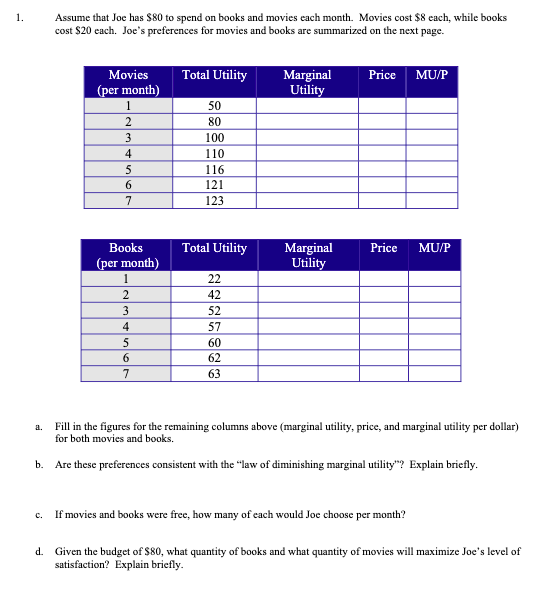### Create an Account

Home / Questions / Assume that Joe has \$80 to spend on books and movies each month. Movies cost \$8 each, whil...

# Assume that Joe has \$80 to spend on books and movies each month. Movies cost \$8 each, while books cost \$20 each. Joe's preferences for movies and books are summarized on the next page. Total Utility

Assume that Joe has \$80 to spend on books and movies each month. Movies cost \$8 each, while books cost \$20 each. Joe's preferences for movies and books are summarized on the next page. Total Utility Price MU/P Marginal Utility Movies (per month) 1 2 3 4 5 6 7 50 80 100 110 116 121 123 Total Utility Price MU/P Marginal Utility Books (per month) 1 2 3 4 5 6 7 22 42 52 57 60 62 63 a. Fill in the figures for the remaining columns above (marginal utility, price, and marginal utility per dollar) for both movies and books. b. Are these preferences consistent with the “law of diminishing marginal utility"? Explain briefly. c. If movies and books were free, how many of each would Joe choose per month? d. Given the budget of \$80, what quantity of books and what quantity of movies will maximize Joe's level of satisfaction? Explain briefly.May 12 2021 View more View LessSubscribe To Get Solution